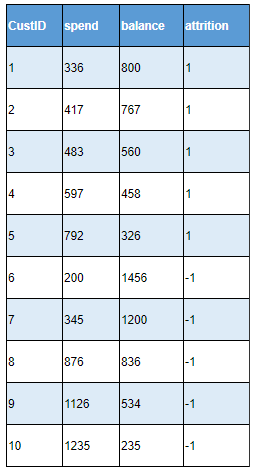Call Us @

(91) 96200 48623

## Blog: Support Vector Machine: Overview

Support Vector Machine (SVM) is one of the machine learning algorithms used for supervised problem sets mainly. Some of the other algorithms which can be explored along with SVM are Decision Tree, Random Forest, Neural Network, or Logistic Regression, specifically for Binary Classification problems.

Due to the mathematical complexity involved in the SVM algorithm, it may be slightly difficult for practitioners to understand the Support Vector Machine.

In this blog, we want to take a simple example and work out the Support Vector Machine algorithm steps. Of course, we will not be able to consider all complexities and details but could be helpful in appreciating the algorithm.

Some of the commonly used phrases in the Support Vector Machine algorithm are

• Support Vectors: Only a few data points (called support vectors) are considered in the SVM algorithm and these data points are called support vectors (value and direction both are important)
• Hyperplane: Plane which separates different classes of the target variable
• Feature space: After the transformation using kernel function, the input support vectors are converted into a feature space which is typically a higher dimensional space than the support vector space
• Kernel function: Transformation functions to make non-separable input space into high dimensional separable space. Some of the commonly available options are linear, polynomial, radial, and sigmoid functions.
• Maximum Margin: In the SVM algorithm, the objective is to find a hyperplane that has maximum margin from support vectors.
• Quadratic Optimization: Objective function in Support Vector Machine formulation is of quadratic type and the optimization framework used for calculating parameters is Quadratic Optimization.  Quadratic Optimization is a well-known area in Optimization.
• Linear and Non-Liner SVM: The problems which require the application of Support Vector Machine could be either Linear and Non-linear formulation.

We will explain some of these in this blog and others in future blogs.

Simple Steps in Linear Support Vector Machine Algorithm

• Get input vector of variables or features and labels (target variable)
• Input vectors are multiplied by another feature space, it is a dot product between these space vectors. This is function is called a kernel.
• A subset of data points or training patterns is selected and these are called support vectors.
• A hyperplane is defined with unknown parameters
• The distance function is formulated between support vectors and the hyperplane.
• Margin is the width between hyperplane and support vectors
• The objective is to find a hyperplane which maximizes the distance between the hyperplane and support vectors

Now let’s consider an example where we want to use SVM based classification model.

Example and context: Credit Card spend and balance indicate customer engagement with the credit card issuer and at the same time indicate profitability (short term and long term) of the bank from the customer.  We have average spending and balance in the last 3 months and want to predict whether customers will attrite or not. So, the response variable is binary, let’s label the customers as 1 and -1. SVM linear classifier can be used to find a hyperplane (in the two-dimensional space, it is a line) that separates target labels 1 and -1.

We are using Linear SVM that can separate between Attrition Label -1 and 1. Also, we will solve it as a simple case and do not use kernel.### Tags

• SOCIAL SHARE :